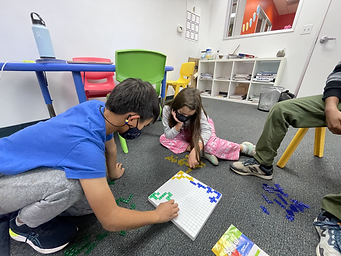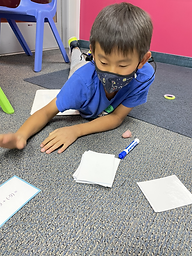## Ms. Caitlyn

### Target 1​

###### Lesson Type:

New

Critical Thinking

:

Spatial Reasoning

Use spatial reasoning to solve problems.

###### 1:

Look at a visual puzzle to analyze the pieces and determine which is the correct piece to choose.

###### 2:

Look at a visual-spatial puzzle to analyze the pieces and determine which is the correct piece to choose.

###### 3:

Identify given relationships between figures and select figures that have an identical relationship.

5th

###### Vocabulary:

Visual-Spatial Reasoning, Four Color Theorem

Activities:

• Students played a spatial reasoning game called Blokus, where they laid down as many colored tiles they could span across a 20 x 20 grid.
• Students could touch the colors of other students, but they could only touch the corners of their own tiles.
• Students applied the Four Color Theorem to visual puzzles, where they needed to color empty spaces such that no two spaces of the same color touched.### Home Exploration

###### Guiding Questions:## Absent Students:

Luca

### Target 2

:

###### 1:

Understand that quantities having a value of more than zero are positive and that quantities having a value of less than zero are negative.

###### 2:

Understand the relationship between positive and negative numbers.

###### 3:

Understand that integers get smaller in value as they move left in order, and larger as they move right in order.

5th

###### Vocabulary:

Positive, Negative, Integer

Activities:

• Students drew cards where they had to solve problems involving the addition/subtraction of integers.
• Students who calculated the higher value got to "steal" the other players cards, and whoever collected the most cards won.
• Students drew five cards and showed how to add and subtract them on the number line.### Home Exploration

###### Guiding Questions:### Target 3

:

###### Vocabulary:

Activities:### Home Exploration